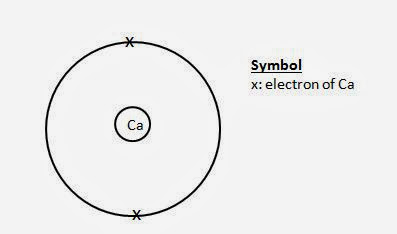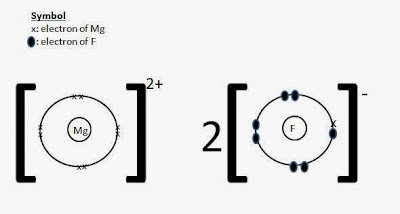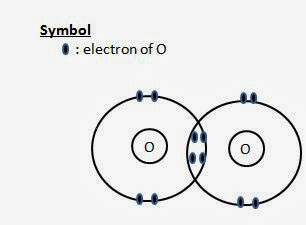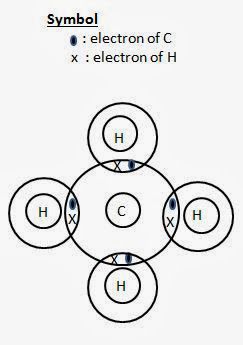Friday, 3 January 2014

Drawing dot- and- cross diagrams, showing the electrons in the outermost shells.

You will need to use the periodic table to determine the Group of the elements.

I will go through this section using examples.

Part 1: Dot- and- cross diagram for atom

Question: Draw a dot- and- cross diagram for oxygen atom, showing the electrons in the outermost shells.

Oxygen is in Group VI --> 6 valence electrons.Dot- and - cross diagram of oxygen atom

Question: Draw a dot- and- cross diagram for calcium atom, showing the electrons in the outermost shells.

Calcium is in Group II --> 2 valence electrons.Dot- and- cross diagram of calcium atom

Part 2: Dot- and- cross diagram for Ionic Compounds

Recap - Ionic compounds are made of metals and non- metals.

Question: Draw a dot- and- cross diagram for calcium oxide, showing the electrons in the outermost shells.

Calcium --> Group II --> 2 valence electrons --> charge is +2, so take away all the valence electrons --> go to the inner shell --> 8 electrons.

Oxygen --> Group VI --> 6 valence electrons --> charge -2, so add 2 electrons --> total 6+2 =8 valence electrons.Dot- and- cross diagram of calcium oxide

Question: Draw a dot- and- cross diagram for magnesium fluoride, showing the electrons in the outermost shells.

Formula of magnesium fluoride is MgF2.

Magnesium--> Group II --> 2 valence electrons --> charge is +2, so take away all the valence electrons --> go to the inner shell --> 8 electrons.

Fluorine --> Group VII --> 7 valence electrons --> charge -1, so add 1 electrons --> total 7+1 =8 valence electrons.Dot- and- cross diagram of magnesium fluoride

Part 3: Dot- and- cross diagrams for simple molecules (covalent molecules).

Recap - Covalent molecules are made up of two of more atoms of non- metals.

Question: Draw a dot- and- cross diagram for oxygen molecule, O2, showing the electrons in the outermost shells.Dot- and- cross diagram of oxygen molecule

Question: Draw a dot- and- cross diagram for chlorine molecule, Cl2showing the electrons in the outermost shells.Dot- and- cross diagram of chlorine

Question: Draw a dot- and- cross diagram for ammonia, NH3, showing the electrons in the outermost shells.Dot- and- cross diagram of ammonia

Question: Draw a dot- and- cross diagram for methane, CH4, showing the electrons in the outermost shells.Dot- and- cross diagram of methane

Questions:
Quizzes and Tests on Chemical Bonding

1 comment:

1.This is a great article for my research. Keep posting. Thank you.

Bob
www.gofastek.com